# How to Calculate and Solve for Total Anomalous Mass | Gravity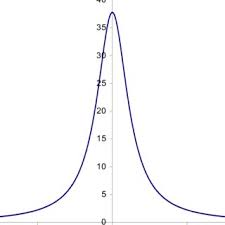The image above represents total anomalous mass.

To compute for total anomalous mass, one essential parameter is needed and this parameter is Number of Area Segments (n).

The formula for calculating total anomalous mass:

ME = 23.9 Σ(ΔgδA)

Where:

ME = Total Anomalous Mass
Δg = Change in Gravity
δA = Area Segment

Let’s solve an example;
Find the total anomalous mass when the number of area of segments is 2 (change of gravity (Δg1) is 5, area segment (δA1) is 12, change of gravity (Δg2) is 8 and area segment (δA2) is 10).

This implies that;

δA1 = Area Segment = 12
Δg1 = Change in Gravity = 5
δA2 = Area Segment = 10
Δg2 = Change in Gravity = 8

Δg δA ΔgδA
5 12 60
8 10 80

Σ(ΔgδA) = 60 + 80
Σ(ΔgδA) = 140

Therefore,

ME = 23.9 Σ(ΔgδA)
ME = 23.9 (140)
ME = 3346

Therefore, the total anomalous mass is 3346 g.

Nickzom Calculator – The Calculator Encyclopedia is capable of calculating the total anomalous mass.

To get the answer and workings of the total anomalous mass using the Nickzom Calculator – The Calculator Encyclopedia. First, you need to obtain the app.

You can get this app via any of these means:

To get access to the professional version via web, you need to register and subscribe for NGN 2,000 per annum to have utter access to all functionalities.
You can also try the demo version via https://www.nickzom.org/calculator

Android (Paid) – https://play.google.com/store/apps/details?id=org.nickzom.nickzomcalculator
Android (Free) – https://play.google.com/store/apps/details?id=com.nickzom.nickzomcalculator
Apple (Paid) – https://itunes.apple.com/us/app/nickzom-calculator/id1331162702?mt=8
Once, you have obtained the calculator encyclopedia app, proceed to the Calculator Map, then click on Geology under Add-on.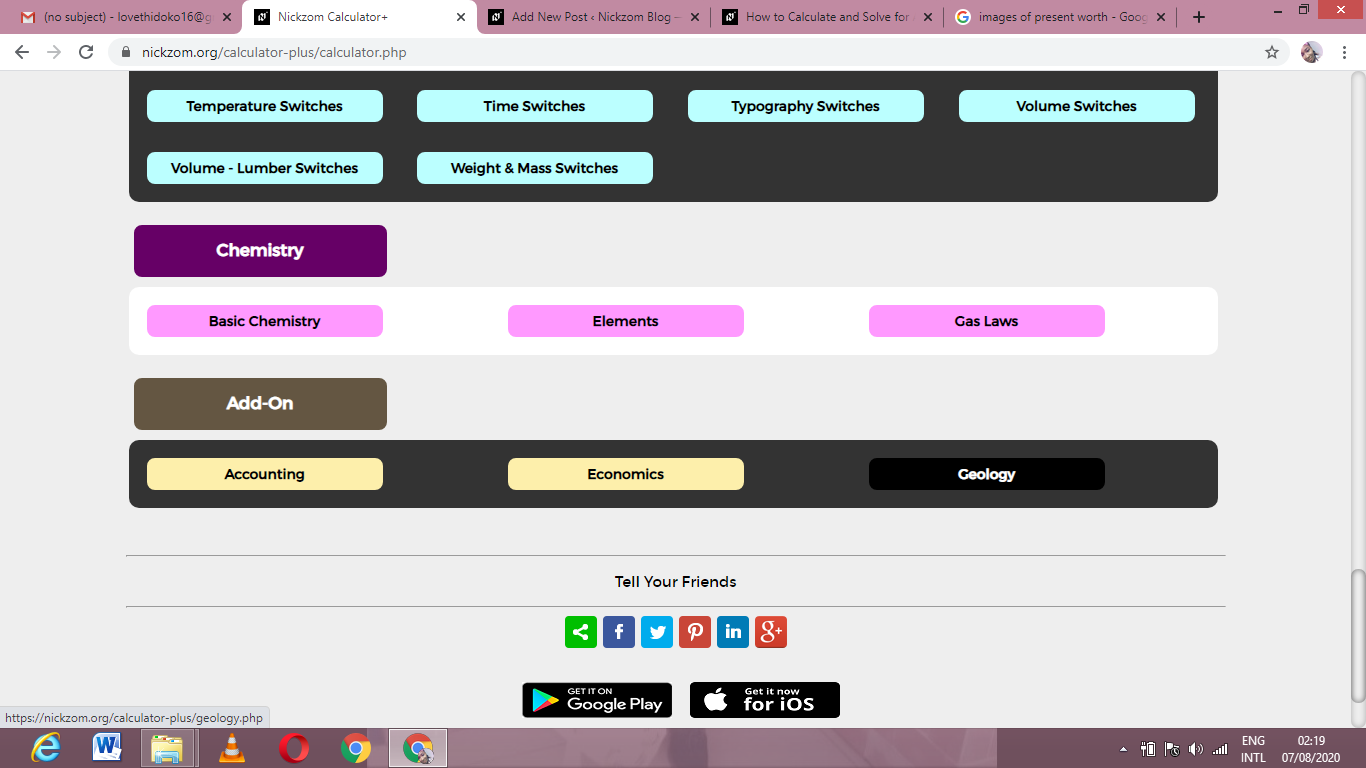Now, Click on Gravity under Geology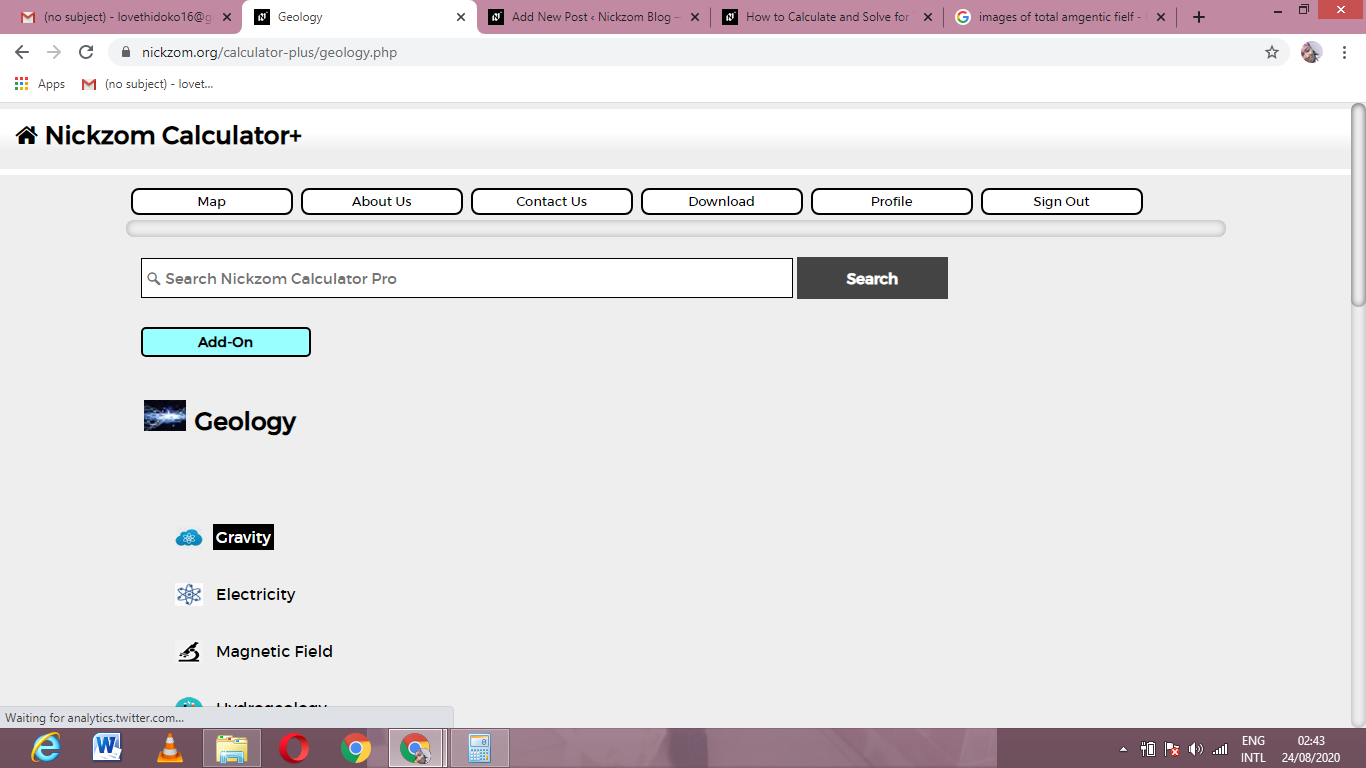Now, Click on Total Anomalous Mass under Gravity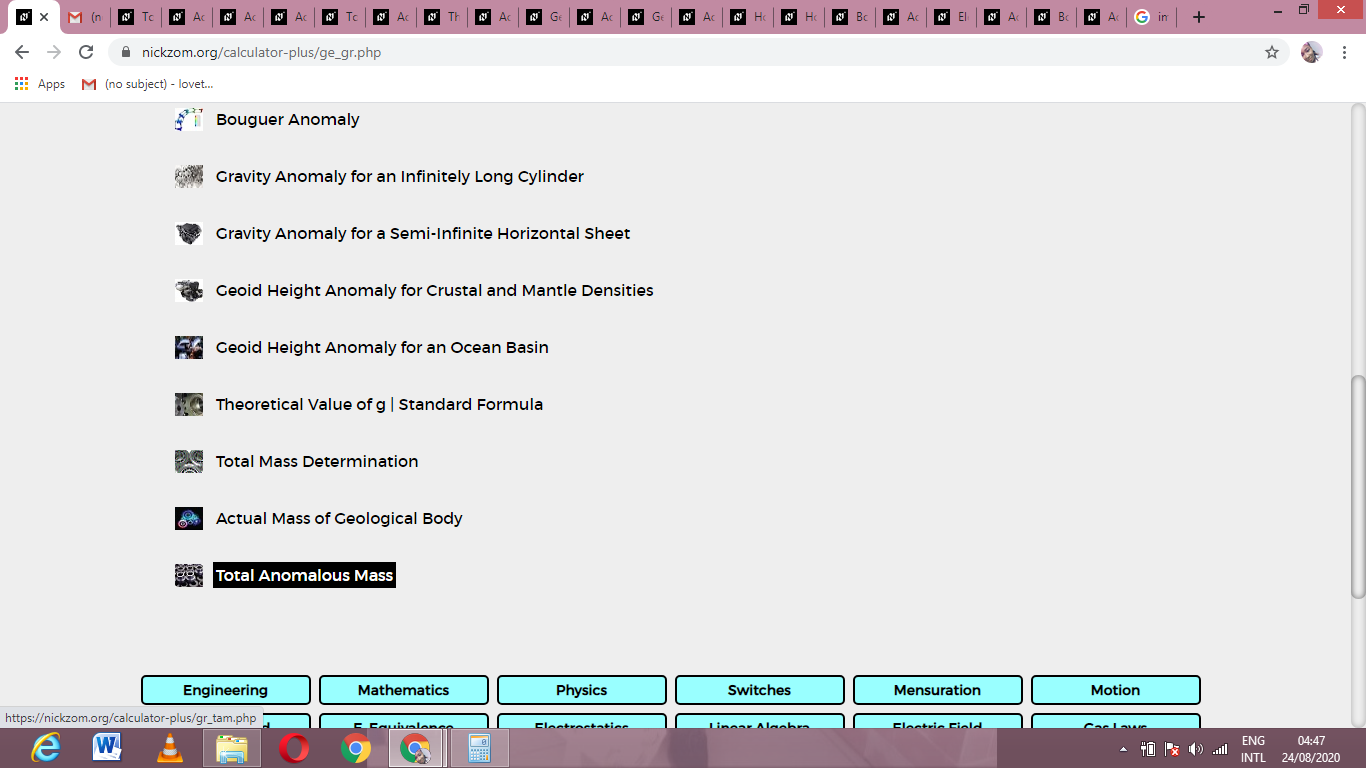The screenshot below displays the page or activity to enter your value, to get the answer for the total anomalous mass according to the respective parameter which is the Number of Area Segments (n).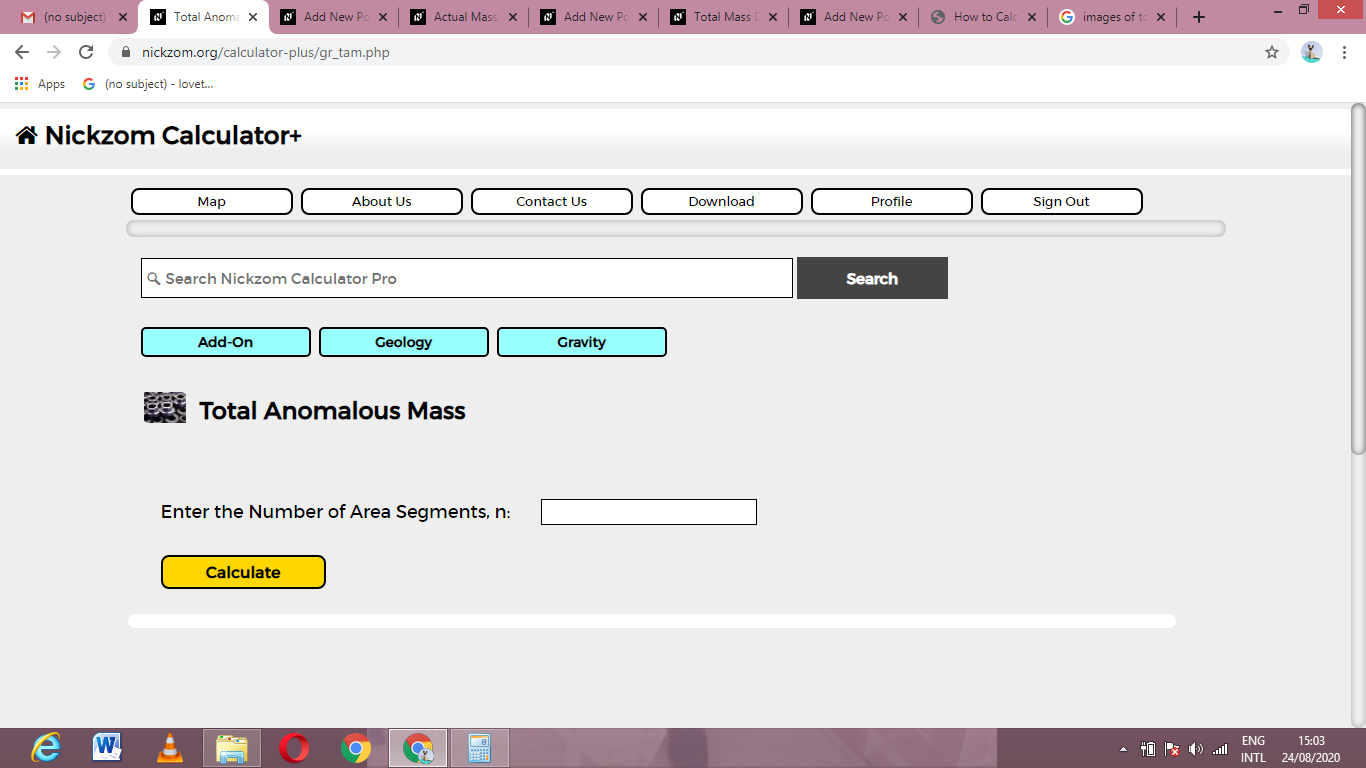Now, enter the value appropriately and accordingly for the parameter as required by the Number of Area Segments (n) is 2.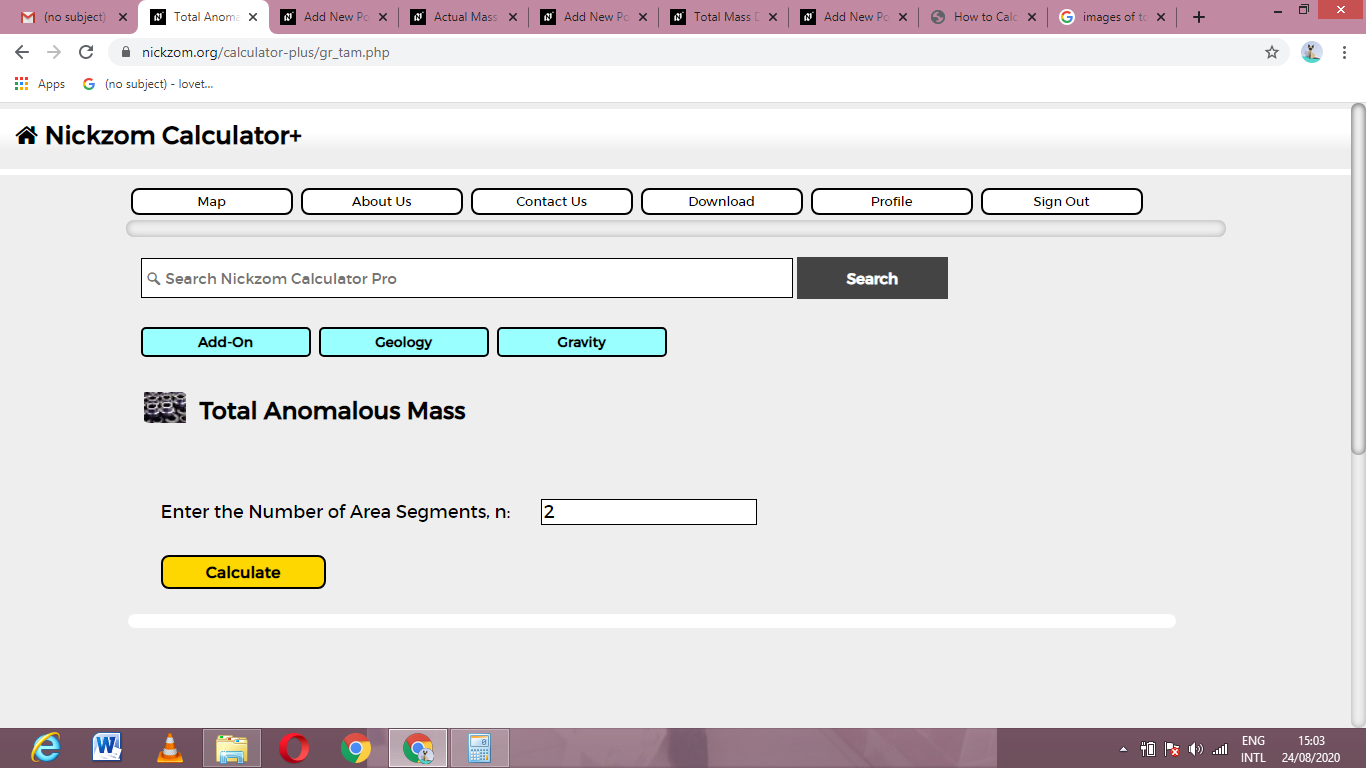Now, enter the values appropriately and accordingly for the parameters as required by the change of gravity (Δg1) is 5, area segment (δA1) is 12, change of gravity (Δg2) is 8 and area segment (δA2) is 10.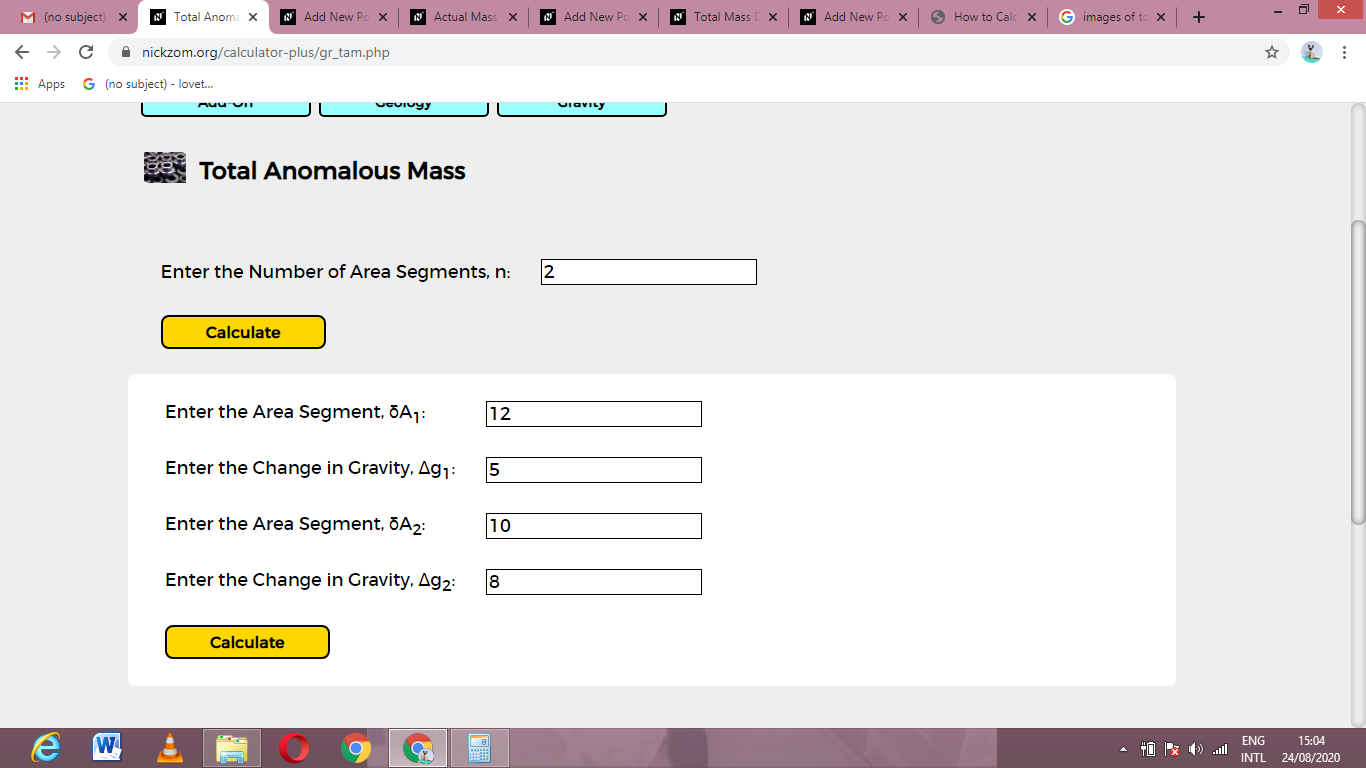Finally, Click on Calculate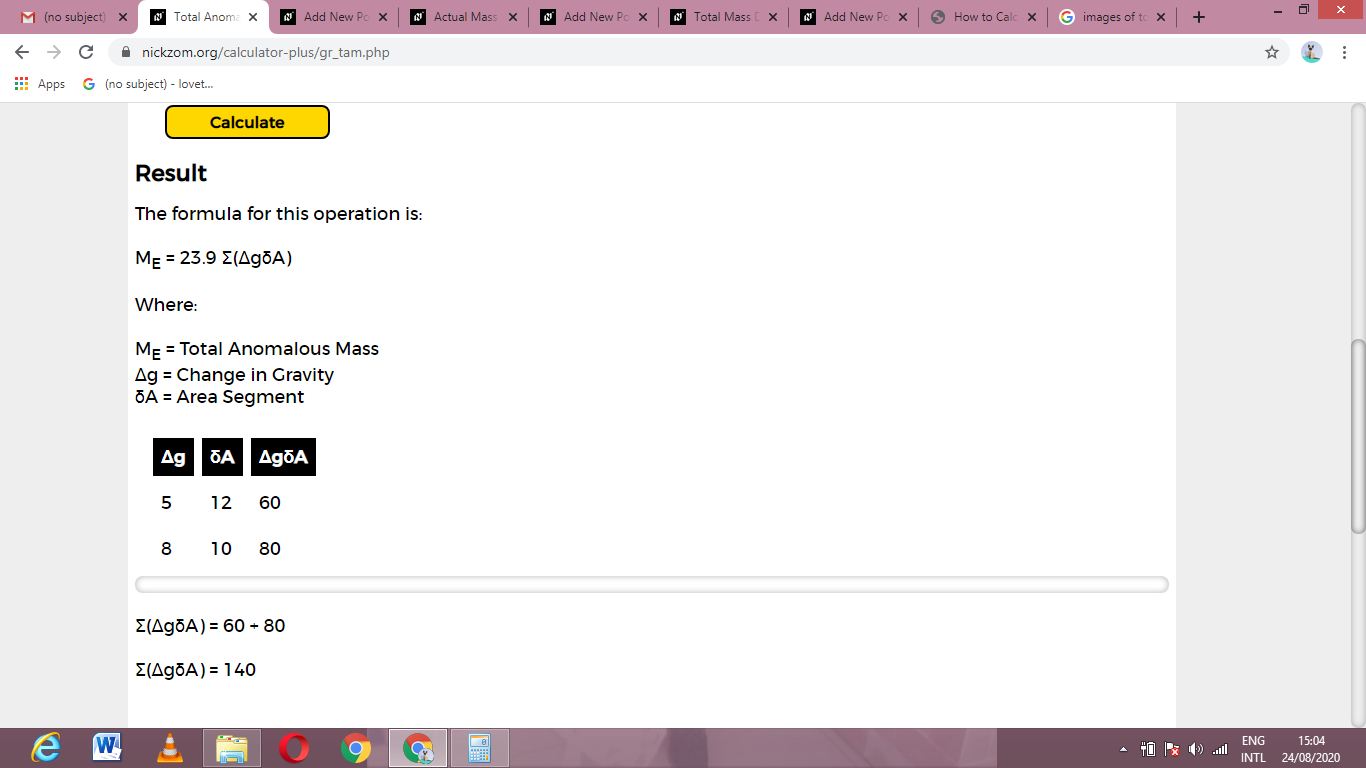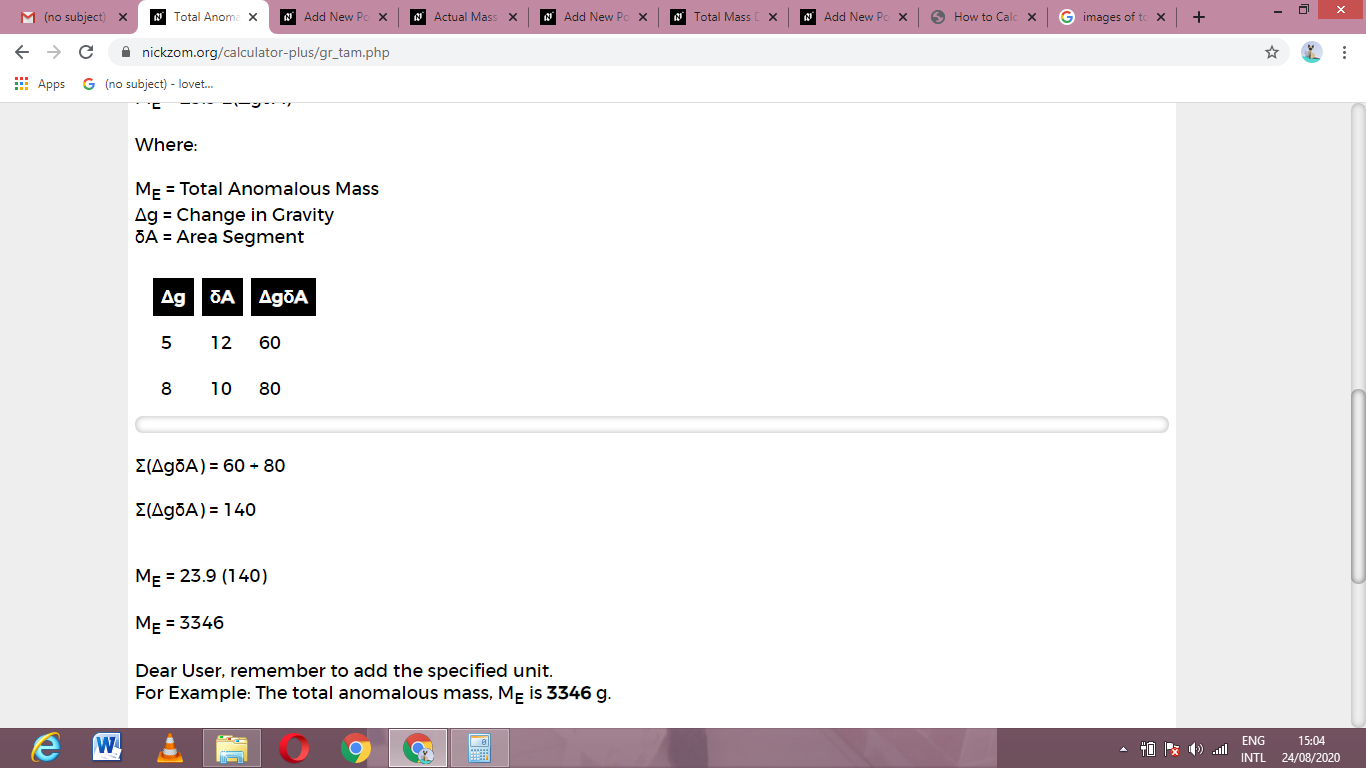As you can see from the screenshot above, Nickzom Calculator– The Calculator Encyclopedia solves for the total anomalous mass and presents the formula, workings and steps too.